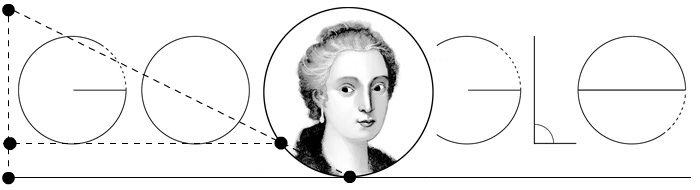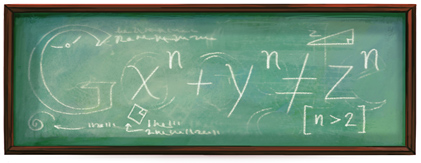# A catenary welcomes you to the beach in OcataPhotography by Bernat Plandolit

Our daily landscape is full of images like this one. Every day we see chains that bar our way and we assume them naturally. However, which shape do these chains take? Can we identify them with known curves? These questions have thrilled some of the greatest mathematicians of all times and have led to the development of elaborated techniques valid today.Johann Bernoulli by J.Rudolf (c.1740)
Source: Wikimedia Commons

The  main characters of this post are the Bernoulli brothers, Jakob (1655-1705) and Johann (1667-1748). They studied together Gottfried W. Leibniz’s works until they mastered it and widened the basis of what is nowadays known as calculus. One of the typical problems was the study of new curves which were created in the 17th century to prove the new differential methods. René Descartes and Pierre de Fermat invented new algebraic and geometrical methods that allowed the study of algebraic curves (those which the coordinates x and y have a polynomial relation). Descartes didn’t consider in his Géométrie curves that were not of this kind, and he called “mechanical curves” to all those ones that are not “algebraic”. In order to study them, he developed non algebraic techniques that allowed the analysis of any kind of curves, either algebraic or mechanical. These mechanical curves had already been introduced a lot of time ago, and were used to solve the three classical problems: squaring the circle, angle trisection and doubling the cube.

When developing calculus, Leibniz’s objective was to develop this general method that Descartes asked for. When Bernoulli brothers started to study curves and its mechanical problems associated, calculus would become its principal solving tool. For instance, in 1690 Jakob solved in Acta Eruditorum, a new problem proposed by Leibniz. In this document, he proved that the problem was equivalent to solve a differential equation and the power of the new technique was also shown.

During the 17th century, mathematicians often proposed problems to the scientific community. The first challenge that Jakob exposed was to find the shape that takes a perfectly flexible and homogeneous chain under the exclusive action of its weight and it is fixed by its ends. This was an old problem that hadn’t been solved yet. As we can see in the picture, the shape taken by the chain is very similar to a parabola and, owing to the fact that it is a well-known curve for centuries, it comes easy to think that it is indeed a parabola (for example, Galileo Galilei thought that he had solved the problem with the parabola). However, in 1646 Christiaan Huygens (1629-1695) was capable to refute it using physical arguments, despite not being able to determine the correct solution.Christiaan Huygens by C.Netscher (1671)
Source: Wikimedia Commons

When Jakob aunched the challenge, Huygens was already 60 years old and successed in finding the curve geometrically, while Johann Bernoulli and Leibniz used the new differential calculus. All of them reached the same result, and Huygens named the curve “catenary”, derived from the latin word “catena” for chain.

Hence the first picture shows a catenary which we observe without paying much attention although all its history. Nowadays, we know it can be described using the hyperbolic cosine, although any of all these great mathematicians couldn’t notice it, as the exponential function had not been introduced yet. Then… How did they do it? Using the natural geometric propreties of the curve so Huygens, Johann Bernoulli and Leibniz could construct it with high precision only usiny geometry. Jakob didn’t know the answer when he proposed the problem and neither found the solution by himself later. So Johann felt proud of himself for surpassing his brother, who had been his tutor (Jakob, who was autodidactic, introduced his younger brother to the world of mathematics while he was studying medicine). What is more, the catenary problem was one of the focusses of their rivalry.

Jakob and Johann were also interested in the resolution of another fmous problem: the braquistochrone. Now, Johann proposed the challenge of finding the trajectory of a particle which travels from one given point to another in the less possible time under the exclusive effect of gravity. In the deadline, only Leibniz had come up with a solution which was sent by letter to Johann with a request of giving more time in order to receive more answers. Johann himself had a solution and in this additional period Jakob, l’Hôpital and an anonymous english author. The answer was a cycloid, a well-known curve since the 1st half of the 17th century. Jakob’s solution was general, developing a tool that was the start of the variational calculus. Johann gave a more imaginative solution based on Fermat’s principle of maximums ans minimums and Snell’s law of refraction of light: he considered a light beam across a medium that changed its refraction index continuously. Given this diference between their minds, Johann enforced his believe that he was better due to his originality and brightness, in contrast with his brother, who was less creative and worked more generally.

A third controversial curve was the tautochrone which is the curve for which the time taken by an object sliding without friction in uniform gravity to its lowest point is independent of its starting point. This property was studied by Huygens and he applied it to the construccion of pendulum clocks.

While we can see catenaries in a park to prevent children from danger or in the entrance to this Catalan beach, we luckily do not see cycloid slides. Thus, children will not descend in the minimum time but will reach the floor safe and sound!

But… who was the misterious english author? For Johann Bernoulli the answer to this question did not involve any mistery: the solution carried inside Isaac Newton’s genius signature -to whom we apologise for having mentioned Leibniz as the calculus inventor-, and he expressed that in the famous sentence:

I recognize the lion by his paw.Isaac Newton by G.Kneller (1702)
Source: Wikimedia Commons

Here we see one more example of a catenary in our daily life:Photography by Bernat Plandolit

This post has been written by Bernat Plandolit and Víctor de la Torre in the subject Història de les Matemàtiques (History of Mathematics, 2014-15).

Location: Ocata beach (map)

# Maria Gaetana Agnesi’s DoodleMaria Gaetana Agnesi (Milan, 16 May, 1718 – 9 January, 1799) was born in a rich Italian family. She was a very clever girl who spoke Italian and French at five years of age and six years later she was able to speak Latin, Greek, Hebrew, German and Spanish. His father was mathematics teachers in the University of Bologna and he never stopped his daughter’s brightness so she wrote a very long speech about women’s rights to be educated when was only 9 y.o. She was always studing and she was very ill one year later because her great interest in all the sciences and humanistic subjects.

She studied Geometry and therefore her father invited her to his meetings with the most learned men in Bologna. Familiar problems let her to retire to study Mathematics and she could learn differential calculus. She became teacher at the university of Bologna and wrote the Instituzioni analitiche ad uso della gioventù italiana (Milan, 1748) where she show a great and didactic introduction to the differential and integral calculus and to the Euler’s works.

The doodle commemorates 296 years since her birthday and we can see the famous Witch of Agnesi. Quoting Wikipedia:

Starting with a fixed circle, a point O on the circle is chosen. For any other point A on the circle, the secant line OA is drawn. The point M is diametrically opposite to O. The line OA intersects the tangent of M at the point N. The line parallel to OM through N, and the line perpendicular to OM through A intersect at P. As the point A is varied, the path of P is the witch.

The curve is asymptotic to the line tangent to the fixed circle through the point O.The Witch was studied by Pierre de Fermat in 1630 and by Guido Grandi in 1703 and Agnesi called it as “versiera” which is Grandi’s name.

# Pierre de Fermat’s doodleMy first post in 2014 is for remembering the Doodle dedicated to Pierre de Fermat’s 410th birthday. It was in Google’s main page in August 17, 2011.

# Christiaan Huygen’s birthdayToday is  Christiaan Huygens’s birthday and this is the doodle dedicated to Huygen’s birthday in April 16, 2009.

Huygens (1629-1695) as one of the first mathematicians to study Probability. He published his De ratiociniis in ludo aleae in 1656 in which he established the foundations of the Calculus of Probabilities after Pascal and Fermat’s letters. Furthermore, he worked on the cycloid, the rectification of curves, the pendulum,…

He also made important contributions to Phisics and Mechanics.Source: Wikimedia Commos

# Fermat’s theorem

Another of the important equations which stands out in the main entrance of Cosmocaixa in Barcelona is Fermat’s last theorem:

Fermat was reading Diophantus’ Arithmetic about the pythagorean triples x2 + y2 = z2 in 1637 when he noticed immediately that n = 2 was the only case which satisfies the equation xn + yn = zn. Then, he wrote in the margin of his edition of Diophantus’ work:

it is impossible to separate a cube into two cubes, or a fourth power into two fourth powers, or in general, any power higher than the second, into two like powers. I have discovered a truly marvelous proof of this, which this margin is too narrow to contain

Probably, Fermat was only able to prove the new theorem for n = 4 using his proof by infinite descent: Fermat proved that there are not three numbers x, y, z such that  x4 + y4 = z2 . First of all, we can suppose that x, y, z are co-prime from (x2)2 + (y2)2 = z2. If <x,y,z> = d, then we can divide all the equation by d2 and we’ll have another equation (x2)2 + (y2)2 = z2 with <x,y,z> = 1.

Now, from the solution of the Pythagorean triples, there are pq such that x2 = 2pq, y2 = p2 – q2 and z = p2 + q2.

We can observe that  y2 = p2 – q2 implies that p2 = q2 – q2 is another Pythagorean triple so there are uv co-prime such that q = 2uvy = u2 – v2 and p = u2 + v2.

So, x2 = 2pq = 2(u2 + v2)(2uv) = 4(uv)(u2 + v2). Since <p,q> = 1, we know that <uv,u2 + v2> = 1 and since their product by 4 is a perfect square, they must be perfect squares too. So there exists a < p such that a2u2 + vp.

Finally, if we had (x2)2 + (y2)2 = z2 , we have obtain p and q co-prime with  z = p2 + q2. Then it is possible to obtain another pair u and v co-prime with p u2 + vand p < z, u < p and v < q. So we can iterate this algorithmic procedure so we’ll obtain a set of pairs of natural numbers each of them lower than the previous and this fact is false, since we’d have an infinite decreasing succession of natural numbers without end!

Nobody accepts that Fermat could have known any other case apart of n = 4! In 1753, Leonhard Euler wrote a letter to Christian Goldbach telling him that he had proved the case n = 3 but the demonstration that he published in his Algebra (1770) was wrong. He tried to find integers p, q, z such that (p2 + 3q2) = zand he found that:

p = a3 – 9ab2q = 3(a2b – b3) ⇒ p2 + 3q2 = (a2 + 3b2)3

He worked with numbers of the form a + b√-3 to find two new numbers a and b less than p and q such that p2 + 3q2 = cube and then he applied the method of infinite descent. Unluckily, he made some mistakes working with the new complex numbers  a + b√-3.

This story is so exciting but, almost quoting Fermat, this post is too short to contain everything. If you are interested in, you must read Simon Singh’s Fermat last theorem which is as interesting as the theorem itself.